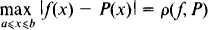# Uniform Approximation

The following article is from The Great Soviet Encyclopedia (1979). It might be outdated or ideologically biased.

## Uniform Approximation

a type of approximation of a function. In uniform approximation, the “distance” on a given set between the given function f(x) and the approximating function P(x) is measured by the least upper bound of the absolute value of their difference. For example, the distance between the continuous function P(x) and the continuous function f(x) on the closed interval [a, b] isUniform approximation is also referred to as Chebyshev approximation, in honor of P. L. Chebyshev, who studied it in 1854. (SeeAPPROXIMATION AND INTERPOLATION OF FUNCTIONS.)

References in periodicals archive ?
Despite of this uniform approximation property, another advantage of the novel class of integrators compared to classical methods is the following: the method converges asymptotically (i.e., c [right arrow] oo) to the numerical method of the corresponding decoupled free Schrodinger limit system (c [right arrow] oo in (1.1)); for details see Section 5.
Their topics include the degree of uniform approximation by generalized discrete singular operators, Voronovskaya-like asymptotic expansion for generalized discrete singular operators, global smoothness preservation and simultaneous approximation by generalized discrete singular operators, the degree of Lp approximation for multivariate generalized discrete singular operators, and the degree of approximation by multivariate complex generalized discrete singular operators.
The system of solutions is shown to be useful for uniform approximation of solutions of initial boundary value problems for (1) as well as for explicit solution of the noncharacteristic Cauchy problem (see ) for (1) in terms of the formal powers arising in the spectral parameter power series (SPPS) method (see [2,3]).
In this paper, we, respectively, establish a polynomial function approximation model for each user's data, since there exists a unique optimal uniform approximation polynomial function for any function in the polynomial space.
Therefore, uniform solutions via the uniform approximation technique are in great need for calculating the solutions in this regime [26,27].
In particular in , linear combinations of moment type operators are considered, which generate sequences of linear operators with a better order of pointwise and uniform approximation. In  special kinds of iterated moment operators were considered and it was remarked that linear combinations of iterated moment operators further improve the order of approximation.
The Carleman's theorem is a pointwise approximation result which generalizes the Weierstrass result on uniform approximation by polynomials in compact intervals, since on any compact subinterval of R, the entire function can in turn be approximated uniformly by polynomials, more exactly by the partial sums of is power series (see Remark 2.9 for the quaternionic setting).
The dotted curves demonstrate some intermediary curves involved in simulations of the uniform approximation process from [W.sub.Lower] to [W.sub.Approx], in a manner that as k decreases from 1/[square root of 0.9] by a small decrement the curves 1 - erf (kz) move uniformly towards numerical solution [W.sub.Num].
In , the optimal piecewise uniform quantizer for that source geometry has been designed assuming four cases for the piecewise uniform approximation of the input radius PDF.
In case the uniform approximation is applied, [c.sub.ik] = 1/2 [[delta].sub.ik] and then

Site: Follow: Share:
Open / Close